# Numerical simulation of fluid-saturated piezoelectric porous media¶

## Mathematical model¶

We consider a porous piezoelectric medium which consists of a piezoelectric matrix, embedded metallic electrodes (conductors) and fluid-filled inclusions. These components are arranged in a periodic lattice so that the medium can be generated by copies of the reference unit cell, see Fig. 1. The mechanical behavior of such a structure can be described using the two-scale asymptotic homogenization method, see [RohanLukes2018].

The mechanical properties of the piezoelectric solid are given by the following constitutive equations

(1)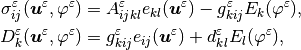where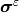is the Cauchy stress tensor,is the electric displacement,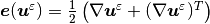is the strain tensor,is the displacement field,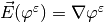is the electric field and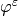is the electric potential. On the right hand side of (1), we have the fourth-order elastic tensor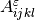(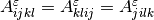), the third-order tensor(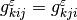), which couples mechanical and electric quantities, and the permeability tensor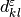. The superscriptdenotes the quantities oscillating within the heterogeneous structure with the period equal to the size of the periodic unit.

The quasi-static problem of the piezoelectric medium is given by the following equilibrium equations

(2)end by the mass conservation equation for the k th fluid inclusion occupying domain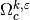(3)where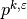is the inclusion pressure andis the fluid compressibility.

## Two-scale homogenization¶Fig. 1 Macroscopic domainand decomposition of microscopic domain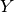We apply the standard homogenization techniques to the above problem. It results in the limit model for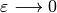, where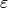is the scale parameter relating the microscopic and macroscopic length scales. The homogenization process leads to local microscopic problems, defined within a reference periodic cell, and to the global problem describing the behavior of the homogenized medium at the macroscopic level. The global problem involves the homogenized material coefficients which are evaluated using the solutions of the local problems. Due to linearity of the problem, the microscopic and macroscopic problems are decoupled.

As we assume given potentials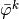in each of the electrode networks, the dielectric properties must be appropriately rescaled in order to preserve the finite electric field in the limit:

(4)The local microscopic responses of the piezoelectric structure are given by the following sub-problems which are solved within the periodic reference cell, see Fig. 1, that is decomposed similarly to the decomposition of domain:

• Find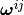,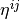such that for all,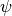and for any(5)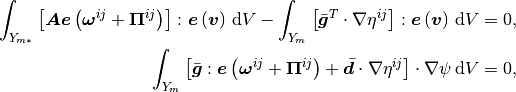where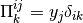.

• Find,such that for all,satisfying

(6)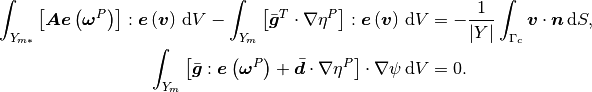• Find,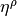such that for all,satisfying

(7)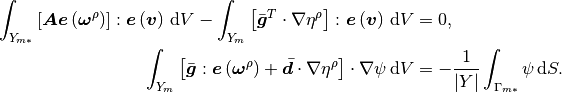• Find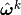,such that for all,and for any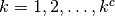(is the number of conductors)

(8)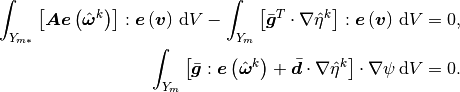The microscopic sub-problems are solved with the periodic boundary conditions andonfor,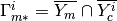is the interface between the matrix part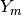and-th conductor. Functions,andare equal to zero on.

With the characteristic responses obtained by solving local sub-problems, the homogenized material coefficients,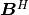,,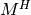andcan be evaluated using the following expressions:

(9)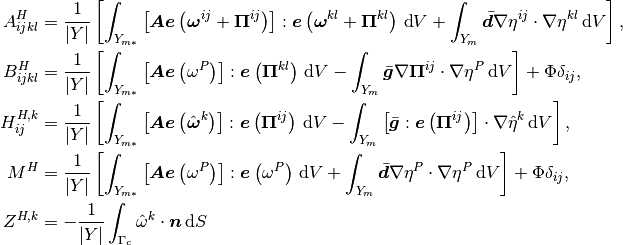The global macroscopic problem is defined in terms of the homogenized coefficients as: Find the macroscopic displacements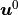and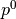such that for alland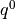(10)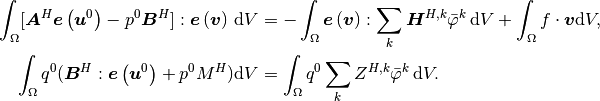We assumed that the volume electric charge is equal to zero, otherwise we would need extra coefficients and right hand side terms.

## Numerical simulation¶

To run the numerical simulation, download the archive, unpack it in the main SfePy directory and type:

./simple.py example_poropiezo-1/poropiezo_macro_dfc.py


This invoke the simply.py script which calculates the macroscopic problem (10) and calls the homogenization engine that solves the local subproblems (5)(6), evaluates the homogenized coefficients (9) and finally performs the reconstruction of the solutions at the microscopic level. See [CimrmanLukesRohan2019] for more details related to the SfePy homogenization engine.

The macroscopic sample is fixed on its left side, so that no displacements are allowed, see Fig. 2 left. The defromation is induced due to piezoelectric effect, as the responce to the prescribed electric potentials,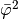, see (10).Fig. 2 Left - boundary conditions applied at the macroscopic level; right - revocered part of the macroscopic sample

The deformed shape of the sample, pressure field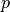and the magnitude of macroscopic strain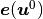are depicted in Fig. 3. The reconstructed strain and electric fields for a givenin the part of the macroscopic domain are shown in Fig. 4.Fig. 3 Deformed macroscopic sample and the resulting fields: left - pressure; right - magnitude of macroscopic strain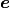Fig. 4 Magnitudes of reconstructed fields: left - strain field; right - electric field